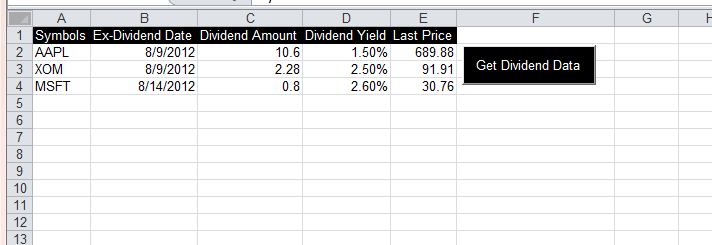*Last updated 9/24/2012

In response to feedback from users in the comments below, the Stock Dividend data downloader has been completely rewritten and and the new file named “DivDates_V2.xls” is available for download here.

The dividend data.

1. Ex Dividend Date
2. Dividend Amount
3. Dividend Yield.
4. Last Price

The instructions are:

1. Enter your symbols in Column A
2. Press the “Get Dividend Data” button.

Below is an screenshot of DivDates_V2.xls.MACD VBA

This post includes links to two example xls files that show both VBA and Formula based calculations for the indicator MACD or Moving Average Convergence Divergence.

Both files contain the exact same set of Open High Low Close data. The formula based version is easier to understand and serves as a way to verify that the VBA code that I wrote is correct. Both methods give the same result.  The major benefit of using VBA is that the parameters for MACD can be easy changed from the input boxes. In addition the VBA method shows only the final result rather than take up five columns.

The VBA based version can be downloaded here.

The formula version can be downloaded here. Below is the code from the VBA based version.

Dim EMAslow As Double, EMAfAst As Double, ws As Worksheet, LR As Integer
Dim eMaF() As Double, eMaS() As Double, EMAdif(), emaPer() As Double, MacDper As Double, coUnt As Integer
Dim DataRange As Range
Dim ExPSlowWeight As Double
Dim ExPFastWeight As Double
Dim PerWeight As Double
'''''''''''''''''''''''''''''''''''''''''''''''''''''''''''''''''''''''''''''''''''''''''''
''  The below three lines are the MACD settings.                                         ''
''  The Values can either be changed here or uncomment the inputbox lines to be prompted.''
'''''''''''''''''''''''''''''''''''''''''''''''''''''''''''''''''''''''''''''''''''''''''''
EMAslow = 13 'InputBox(Prompt:="Enter Macd Slow settings.", Title:="MACD SLOW", Default:="13")
EMAfAst = 5 'InputBox(Prompt:="Enter Macd Fast settings.", Title:="MACD Fast", Default:="5")
MacDper = 6 'InputBox(Prompt:="Enter Macd Period settings.", Title:="MACD Period", Default:="6")
ExPSlowWeight = 2 / (EMAslow + 1)
PerWeight = 2 / (MacDper + 1)
ExPFastWeight = 2 / (EMAfAst + 1)
Set ws = ThisWorkbook.Worksheets("VBA") 'or use exact sheet name for example ThisWorkbook.worksheet("Sheet1")
'slow
With ws
LR = .Cells(Rows.coUnt, "A").End(xlUp).Row
For Each DataRange In ws.Range(.Cells(2, "A"), .Cells(LR, "A"))
coUnt = DataRange.Row + 1
'fill the eMA slow Array
ReDim Preserve eMaS(1 To coUnt)
If coUnt = EMAslow + 1 Then
'get the first value which is the Simple Moving average
eMaS(coUnt) = Application.Average(ws.Range(.Cells(2, "E"), .Cells(coUnt, "E")))
ElseIf coUnt > EMAslow Then
eMaS(coUnt) = (.Cells(coUnt, "E") * ExPSlowWeight) + (eMaS(coUnt - 1) * (1 - ExPSlowWeight))
End If
Next DataRange
'fast
For Each DataRange In ws.Range(.Cells(2, "A"), .Cells(LR, "A"))
coUnt = DataRange.Row + 1
'fill the eMA slow Array
ReDim Preserve eMaF(1 To coUnt)
If coUnt = EMAfAst + 1 Then
'get the first value which is the Simple Moving average
eMaF(coUnt) = Application.Average(ws.Range(.Cells(2, "E"), .Cells(coUnt, "E")))
ElseIf coUnt > EMAfAst Then
eMaF(coUnt) = (.Cells(coUnt, "E") * ExPFastWeight) + (eMaF(coUnt - 1) * (1 - ExPFastWeight))
End If
Next DataRange
ReDim Preserve EMAdif(EMAslow To UBound(eMaF))
For coUnt = EMAslow + 1 To UBound(eMaF)
EMAdif(coUnt) = eMaF(coUnt) - eMaS(coUnt)
Next coUnt
'MacD Period
Dim x As Integer, y As Integer, z As Integer, Avee As Double
y = EMAslow + MacDper - 1
For x = y To UBound(EMAdif) - 2
'get the SMA for first value
If x = y Then
For z = EMAslow + 1 To EMAslow + MacDper  '(EMAslow + MacDper - 1)
Ave = Ave + EMAdif(z)
Next z
ReDim emaPer(x To LR)
emaPer(x) = Ave / MacDper
ElseIf x > y Then
emaPer(x) = (EMAdif(x + 1) * PerWeight) + (emaPer(x - 1) * (1 - PerWeight))
End If
Next x
x = Empty: y = Empty: z = Empty
x = LBound(emaPer)
y = UBound(emaPer)
For z = x To y - 1
.Cells(z + 1, "J") = EMAdif(z + 1) - emaPer(z)
Next z
End With

Data Converter to Convert OHLC Data to a larger timeframe.

If you have ever wanted to take your existing Open High Low Close historical trading data and convert it into larger time frame, the following Excel VBA based data converter I wrote may do the trick.DataConverter.xls allows you to configure the Number of OHLC bars that should be condensed down into a single bar. You also can list the file name, sheet, and columns where your data is located.  The data will almost instantly be transformed into the higher time frame you have requested.

Instructions: